# 2016 Halloween Laser Maze

Experience the challenge and thrill of deactivating the system without breaking a laser beam. Built with Arduino, BLE, and DMX lighting.

IntermediateShowcase (no instructions)14 days14,713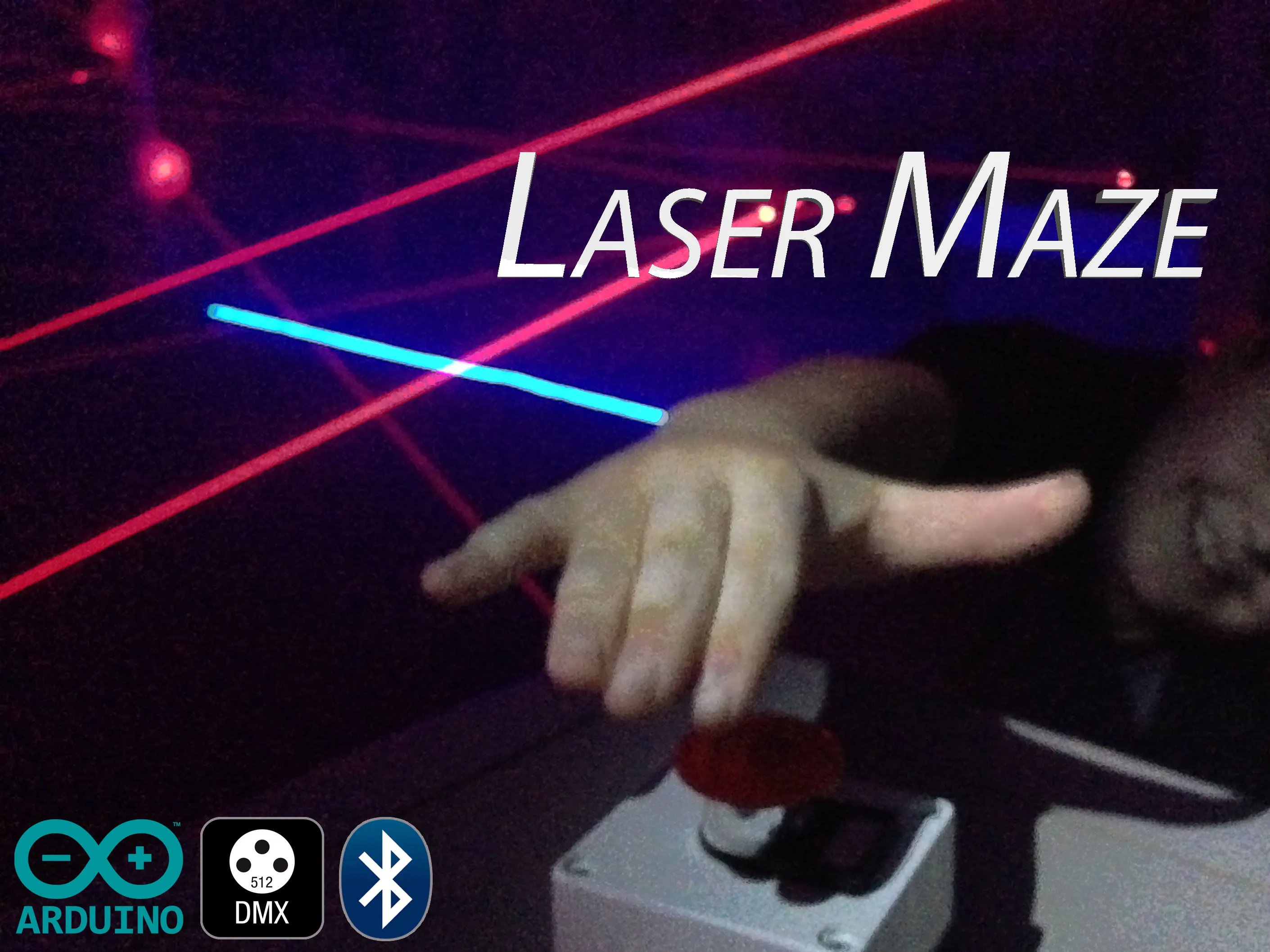## Things used in this project

### Hardware components

 Laser Module
×15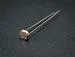Photo resistor
×15Arduino UNO & Genuino UNO
×1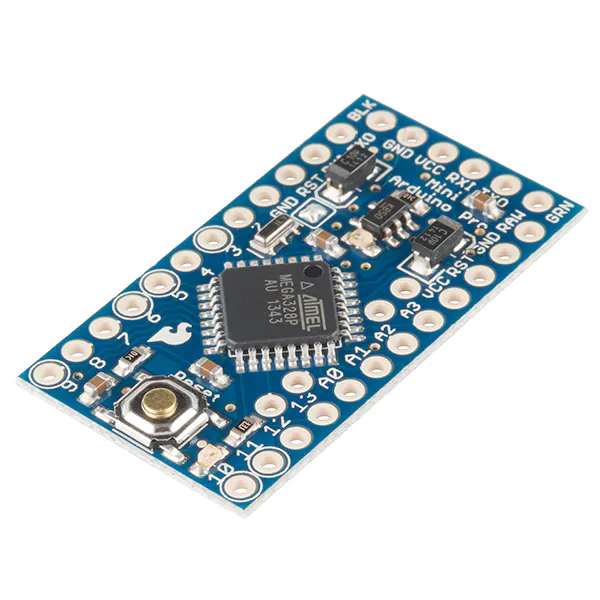SparkFun Arduino Pro Mini 328 - 5V/16MHz
×1
 DMX Shield
×1
 RedBear BLE Shield
×1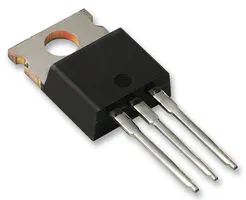Darlington High Power Transistor Used to power lasers.
×1
 5M RGB LED Strip
×2
 22 AWG Hook-Up Wire
×1
 3 Channel DMX Decoder
×1
 Block Terminal Strip
×1
 Cat5 Cable Used to connect laser / sensor towers to central hub.
×1
 Barrel Jack Screw Terminal Connector (M+F Set)
×30
 Scrap Wood Used to build laser pan/tilt brackets and laser / sensor towers.
×1
 Emergency Stop Button
×1
 Plastic Food Container Used with diffused plastic wrap to create sensor housings.
×15
 Fog machine
×1

### Software apps and online services

 Arduino Manager

### Hand tools and fabrication machinesSoldering iron (generic)
 Table Saw
 Drill

## Schematics

### Schematic

Note: Shields are not shown in this representation. BLE shield is stacked on Arduino Uno. DMX shield pins are connected to Arduino Pro Mini. Also, an LED is the placeholder for lasers. This image shows the series wiring of the photoresistors, even though they are not actually on the breadboard.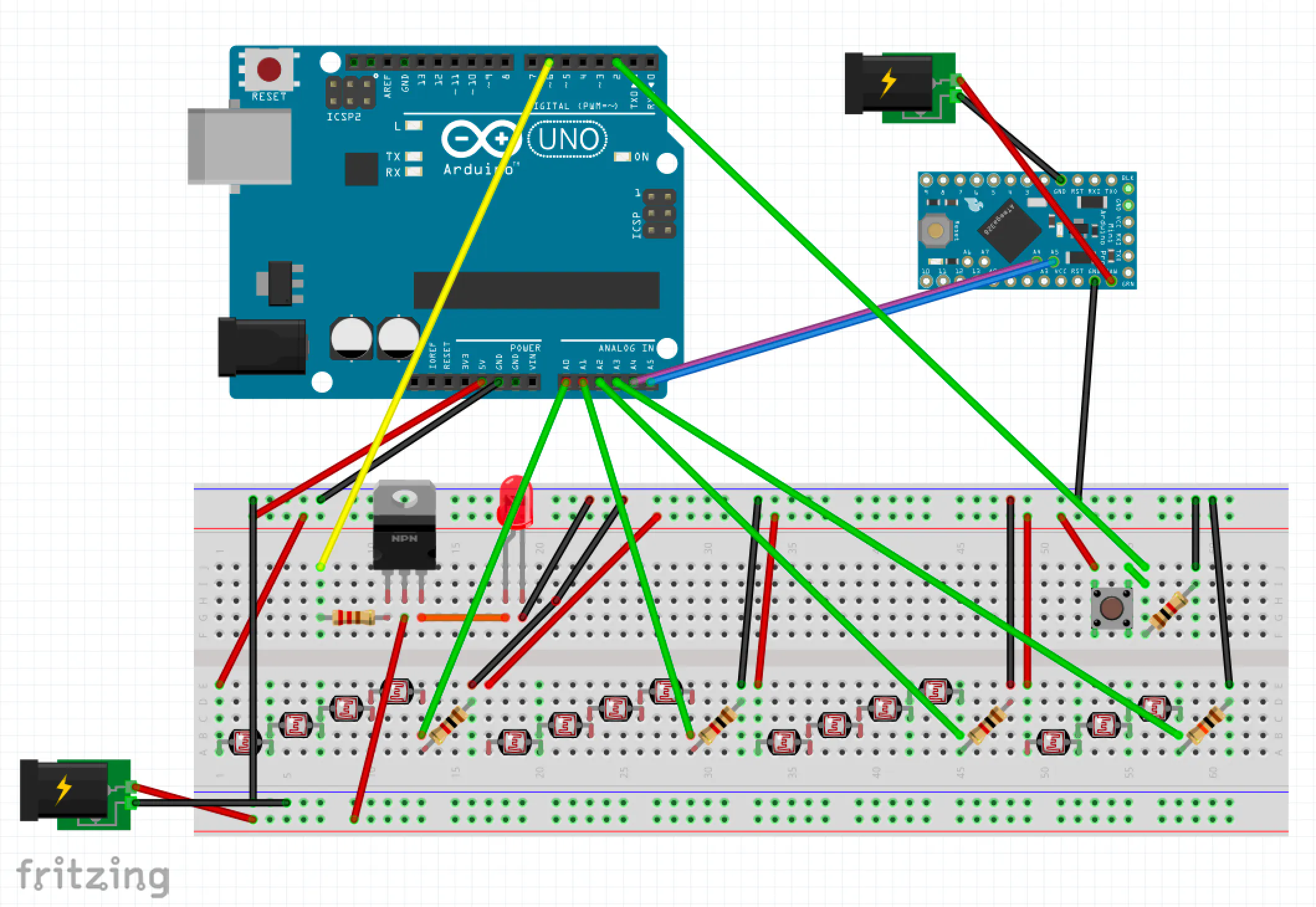## Code

### Master (Arduino Uno)

Arduino
Arduino Uno with BLE Shield processes sensor data, laser state, button state, and Arduino Manager.
```/*2016 Halloween Laser Maze created by Sam Horne.
*
* Note: This sketch is a work in progress and contains a few redundant variables and declarations.
* While it worked correctly for the maze, I will definetely improve it in the future.
*/

//#include <RBL_nRF8001.h>
//#include <SPI.h>
//#include <boards.h>
#include <IOSControllerBLE.h>  //Initialize Arduino Manager library.
#include <Wire.h>  //Initialize Wire library to connect both Arduinos.

#define LASERPIN      6     //Lasers connected with transistor to Digital pin6.
#define SENSOR0           0     //Four sensor groups, each containing photoresistors wired in series
#define SENSOR1           2
#define SENSOR2           1
#define SENSOR3           3

boolean laservalue;    //State of laser
int buttonPin = 2;     //Stop button
int buttonState = 0;
//Sensor variables.
int S0;
int S1;
int S2;
int S3;
//Threshold variables
int T0;
int T1;
int T2;
int T3;
int sound; //Variable controls audio widget in Arduino Manager
int aim;  //Allows lights and alarm to be disabled from Arduino Manager
int laserState;
int LASER = 0;
byte x = 0;  //For wire library transmission

IOSControllerBLE iosController(&doWork,&doSync,&processIncomingMessages,&processOutgoingMessages,&processAlarms,&deviceConnected,&deviceDisconnected);  //Necessary for Arduino Manager

void setup()
{
ble_begin();
Wire.begin();
Serial.begin(115200);

//  pinMode(CONNECTIONPIN,OUTPUT);
pinMode(buttonPin , INPUT);  //Stop button
pinMode(LASERPIN,OUTPUT);  //Laser
pinMode(SENSOR0, INPUT);
pinMode(SENSOR1,INPUT);
pinMode(SENSOR2, INPUT);
pinMode(SENSOR3, INPUT);

laservalue = 0;  /Set the laser value.
digitalWrite(LASERPIN,laservalue);  //Set the laser value.
}

void loop() {
iosController.loop();
if(buttonState == HIGH) {         //User presses stop button to win game.
Wire.beginTransmission(9); //Begin transmitting on channel 9.
Wire.write(9);  //Send a single byte (9) which triggers the win sequence on the Pro Mini.
Wire.endTransmission();
LASER = LOW;   //Laser turns off if someone wins.
aim = HIGH;  //This prevents the alarm from sounding when the sensor values drop due to the inactive laser.
iosController.writeMessage("aim", HIGH);  //Sync switch positions.
digitalWrite(LASERPIN,LOW);  //Laser turns off if someone wins.
iosController.writeMessage("laserState", LOW); //Sync switch positions.
delay(1000);
}
if (S0 < T0 && aim == 0)  {  //When sensor value drops below threshold and aim mode is not enabled.
sound = 1;  //Sound the alarm
x = 0;
Wire.beginTransmission(9);
Wire.write(0);  //Send value 0 to Pro Mini to trigger flashing red lights.
Wire.endTransmission();
digitalWrite(LASERPIN,LOW); //Turn the laser off.
}
else if (S1 < T1 && aim == 0) {  //When sensor value drops below threshold and aim mode is not enabled.
sound = 1;  //Sound the alarm
x = 0;
Wire.beginTransmission(9);
Wire.write(0);  //Send value 0 to Pro Mini to trigger flashing red lights.
Wire.endTransmission();
digitalWrite(LASERPIN,LOW); //Turn the laser off.
}
else if (S2 < T2 && aim == 0) {  //When sensor value drops below threshold and aim mode is not enabled.
sound = 1;  //Sound the alarm
x = 0;
Wire.beginTransmission(9);
Wire.write(0);  //Send value 0 to Pro Mini to trigger flashing red lights.
Wire.endTransmission();
digitalWrite(LASERPIN,LOW); //Turn the laser off.
}
else if (S3 < T3 && aim == 0) {  //When sensor value drops below threshold and aim mode is not enabled.
sound = 1;  //Sound the alarm
x = 0;
Wire.beginTransmission(9);
Wire.write(0);  //Send value 0 to Pro Mini to trigger flashing red lights.
Wire.endTransmission();
digitalWrite(LASERPIN,LOW); //Turn the laser off.
}
else {
sound = 0; //If no sensor events occur, no sound will happen.
}

}

void doWork() {
//Read the sensor group values from the analog pins.
delay(5);
delay(5);
delay(5);
delay(5);
iosController.writeMessage("S0",S0);

}

void doSync (char *variable) {
}

void processIncomingMessages(char *variable, char *value) {

if (strcmp(variable,"LASER")==0) { //Process incoming messages from Arduino Manager

laservalue = atoi(value);
laserState = atoi(value);

digitalWrite(LASERPIN,laservalue);  //If LASER switch goes HIGH, turn on laser
}

if (strcmp(variable,"T0")==0) {

T0 = atoi(value);
}
if (strcmp(variable,"T1")==0) {

T1 = atoi(value);
}
if (strcmp(variable,"T2")==0) {

T2 = atoi(value);
}
if (strcmp(variable,"T3")==0) {

T3 = atoi(value);
}
if (strcmp(variable,"aim")==0) {  //Sync aim value.
aim = atoi(value);
}

}

void processOutgoingMessages() {

//Sync sensor values to Arduino Manager.
iosController.writeMessage("S0",S0);
iosController.writeMessage("S1",S1);
iosController.writeMessage("S2",S2);
iosController.writeMessage("S3",S3);
iosController.writeMessage("sound", sound);
iosController.writeMessage("x",x);
}
void deviceConnected () {
}

void deviceDisconnected () {
}

#ifdef ALARMS_SUPPORT

void processAlarms(char *alarm) {

iosController.log("Alarm fired: ");
iosController.logLn(alarm);

}
#endif

float getVoltage(int pin) {

return (analogRead(pin) * .004882813);  // converting from a 0 to 1023 digital range
// to 0 to 5 volts (each 1 reading equals ~ 5 millivolts
}
```

### Slave (Arduino Pro Mini)

Arduino
Arduino Pro Mini with DMX shield reads I2C commands from Arduino Uno and controls lighting devices.
This code is not essential to the project, and the project can function without the second Arduino (lighting).
```#include <DmxSimple.h>

#include <Wire.h>
#include <wiring.h>
int DE = 2;  //DMX enable
int x = 0; //Wire transmission variable
int v = 15; // brightness value

void setup() {
/* The most common pin for DMX output is pin 3, which DmxSimple
** uses by default. If you need to change that, do it here. */
DmxSimple.usePin(4);
pinMode(DE, OUTPUT);
digitalWrite(DE, HIGH); //DMX enable
DmxSimple.write(1, 0);  //Startup scene
DmxSimple.write(2, 0);
DmxSimple.write(3, 0);
DmxSimple.write(27, 10);
Wire.begin(9);
Serial.begin(115200);

DmxSimple.maxChannel(27);
}

}

void loop() {
Serial.println(x);
if (x == 9) {  //Flashing green sequence
DmxSimple.write(6, 0);
DmxSimple.write(18, 0);
DmxSimple.write(15, 0);
DmxSimple.write(27, 0);
DmxSimple.write(5, 255);
DmxSimple.write(8, 255);
DmxSimple.write(11, 255);
DmxSimple.write(14, 255);
DmxSimple.write(17, 255);
DmxSimple.write(20, 255);
DmxSimple.write(23, 255);
DmxSimple.write(26, 255);
DmxSimple.write(41, 255);
delay(100);
DmxSimple.write(5, 0);
DmxSimple.write(8, 0);
DmxSimple.write(11, 0);
DmxSimple.write(14, 0);
DmxSimple.write(17, 0);
DmxSimple.write(20, 0);
DmxSimple.write(23, 0);
DmxSimple.write(26, 0);
DmxSimple.write(41, 0);
delay(100);
}
if (x == 0) { //Flashing red sequence.
DmxSimple.write(6, 0);
DmxSimple.write(9, 0);
DmxSimple.write(12, 0);
DmxSimple.write(15, 0);
DmxSimple.write(18, 0);
DmxSimple.write(21, 0);
DmxSimple.write(24, 0);
DmxSimple.write(27, 0);
DmxSimple.write(42, 0);
DmxSimple.write(4, 255);
DmxSimple.write(7, 255);
DmxSimple.write(10, 255);
DmxSimple.write(13, 255);
DmxSimple.write(16, 255);
DmxSimple.write(19, 255);
DmxSimple.write(22, 255);
DmxSimple.write(25, 255);
DmxSimple.write(40, 255);
delay(100);
DmxSimple.write(4, 0);
DmxSimple.write(7, 0);
DmxSimple.write(10, 0);
DmxSimple.write(13, 0);
DmxSimple.write(16, 0);
DmxSimple.write(19, 0);
DmxSimple.write(22, 0);
DmxSimple.write(25, 0);
DmxSimple.write(40, 0);
delay(100);
}
delay(10);
}
```

## Credits

### Sam Horne

0 projects • 6 followers
Former Electrical Engineering Intern at Walt Disney World Resort. Student at University of Memphis.